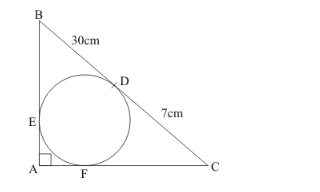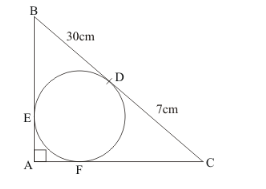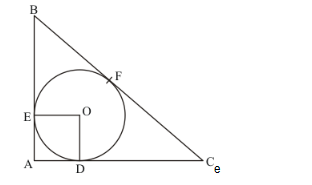# In the given figure, BDC is a tangent to the given circle at point D such that BD = 30 cm and CD = 7 cm.

Question:

In the given figure, BDC is a tangent to the given circle at point D such that BD = 30 cm and CD = 7 cm. The other tangents BE and CF are drawn respectively from B and C to the circle and meet when produced at A making BAC a right angle triangle. Calculate (i) AF (ii) radius of the circle.Solution:

The given figure is below(i) The given triangle ABC is a right triangle where side BC is the hypotenuse. Let us now apply Pythagoras theorem. We have,

$A B^{2}+A C^{2}=B C^{2}$

Looking at the figure we can rewrite the above equation as follows.

$(B E+E A)^{2}+(A F+F C)^{2}=(30+7)^{2} \ldots \ldots(1)$

From the property of tangents we know that the length of two tangents drawn from the same external point will be equal. Therefore we have the following,

BE = BD

It is given that BD = 30 cm. Therefore,

BE = 30 cm

Similarly,

CD = FC

It is given that CD = 7 cm. Therefore,

FC = 7 cm

Also, on the same lines,

EA = AF

Let us substitute these in equation (1). We get,

$(B E+E A)^{2}+(A F+F C)^{2}=(30+7)^{2}$

$(30+A F)^{2}+(A F+7)^{2}=37^{2}$

$\left(30^{2}+2 \times 30 \times A F+A F^{2}\right)+\left(A F^{2}+2 \times 7 \times A F+7^{2}\right)=1369$

$900+60 A F+A F^{2}+A F^{2}+14 A F+49=1369$

$2 F^{2}+74 A F-420=0$

$A F^{2}+37 A F-210=0$

$A F^{2}+42 A F-5 A F-210=0$

$A F(A F+42)-5(A F+42)=0$

$(A F-5)(A F+42)=0$

$(A F-5)(A F+42)=0$

Therefore,

AF = 5

Or,

AF = − 42

Since length cannot have a negative value,

AF = 5

(ii) Let us join the point of contact E with the centre of the circle say O. Also, let us join the point of contact F with the centre of the circle O. Now we have a quadrilateral AEOF.'(Given in the problem)(Since the radius will always be perpendicular to the tangent at the point of contact)(Since the radius will always be perpendicular to the tangent at the point of contact)

We know that the sum of all angles of a quadrilateral will be equal to. Therefore,

$\angle E A D+\angle O D A+\angle E O D+\angle O E A=360^{\circ}$

$90^{\circ}+90^{\circ}+90^{\circ}+\angle E O D=360^{\circ}$

$\angle E O D=90^{\circ}$

Since all the angles of the quadrilateral are equal to 90° and the adjacent sides are equal, this quadrilateral is a square. Therefore all the sides are equal. We have found that

AF = 5

Therefore,

OD = 5

OD is nothing but the radius of the circle.

Thus we have found that AF = 5 cm and radius of the circle is 5 cm.